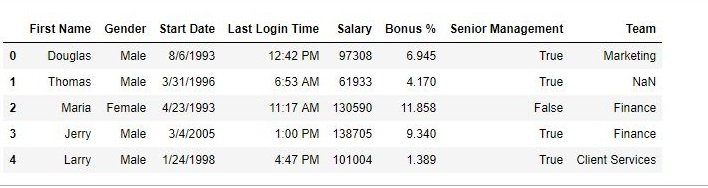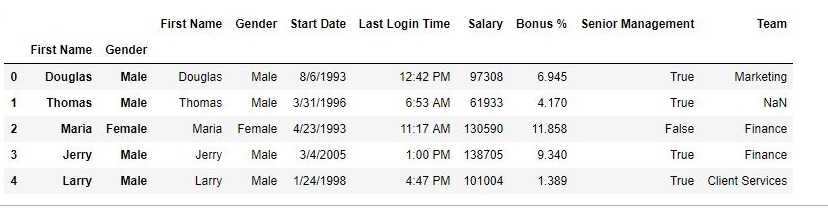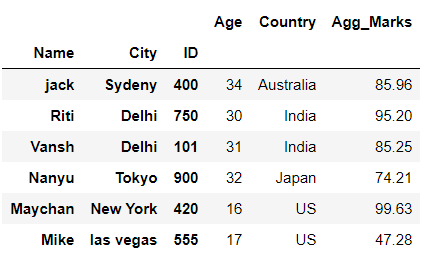Related Articles
Python | Pandas DataFrame.set_index()
• Last Updated : 01 Sep, 2020

Python is a great language for doing data analysis, primarily because of the fantastic ecosystem of data-centric python packages. Pandas is one of those packages and makes importing and analyzing data much easier.
Pandas set_index() is a method to set a List, Series or Data frame as index of a Data Frame. Index column can be set while making a data frame too. But sometimes a data frame is made out of two or more data frames and hence later index can be changed using this method.
Syntax:

DataFrame.set_index(keys, drop=True, append=False, inplace=False, verify_integrity=False)

Parameters:

keys: Column name or list of column name.
drop: Boolean value which drops the column used for index if True.
append: Appends the column to existing index column if True.
inplace: Makes the changes in the dataframe if True.
verify_integrity: Checks the new index column for duplicates if True.

To download the CSV file used, Click Here.
Code #1: Changing Index column
In this example, First Name column has been made the index column of Data Frame.

## Python3

 `# importing pandas package``import` `pandas as pd` `# making data frame from csv file``data ``=` `pd.read_csv(``"employees.csv"``)` `# setting first name as index column``data.set_index(``"First Name"``, inplace ``=` `True``)` `# display``data.head()`

Output:
As shown in the output images, earlier the index column was a series of number but later it has been replaced with First name.
Before operation –After operation –Code #2: Multiple index Column
In this example, two columns will be made as index column. Drop parameter is used to Drop the column and append parameter is used to append passed columns to the already existing index column.

## Python3

 `# importing pandas package``import` `pandas as pd` `# making data frame from csv file``data ``=` `pd.read_csv(``"employees.csv"``)` `# setting first name as index column``data.set_index([``"First Name"``, ``"Gender"``], inplace ``=` `True``,``                            ``append ``=` `True``, drop ``=` `False``)` `# display``data.head()`

Output:
As shown in the output Image, the data is having 3 index columns.Code #3: Setting a single Float column as Index in Pandas DataFrame

## Python3

 `# importing pandas library``import` `pandas as pd` `# creating and initializing a nested list``students ``=` `[[``'jack'``, ``34``, ``'Sydeny'``, ``'Australia'``,``85.96``],``            ``[``'Riti'``, ``30``, ``'Delhi'``, ``'India'``,``95.20``],``            ``[``'Vansh'``, ``31``, ``'Delhi'``, ``'India'``,``85.25``],``            ``[``'Nanyu'``, ``32``, ``'Tokyo'``, ``'Japan'``,``74.21``],``            ``[``'Maychan'``, ``16``, ``'New York'``, ``'US'``,``99.63``],``            ``[``'Mike'``, ``17``, ``'las vegas'``, ``'US'``,``47.28``]]` `# Create a DataFrame object``df ``=` `pd.DataFrame(students,``                      ``columns``=``[``'Name'``, ``'Age'``, ``'City'``, ``'Country'``,``'Agg_Marks'``],``                           ``index``=``[``'a'``, ``'b'``, ``'c'``, ``'d'``, ``'e'``, ``'f'``])` `# here we set Float column 'Agg_Marks' as index of data frame``# using dataframe.set_index() function``df ``=` `df.set_index(``'Agg_Marks'``)`  `# Displaying the Data frame``df`

Output :In the above example, we set the column ‘Agg_Marks‘ as an index of the data frame.

Code #4: Setting three columns as MultiIndex in Pandas DataFrame

## Python3

 `# importing pandas library``import` `pandas as pd` `# creating and initializing a nested list``students ``=` `[[``'jack'``, ``34``, ``'Sydeny'``, ``'Australia'``,``85.96``,``400``],``            ``[``'Riti'``, ``30``, ``'Delhi'``, ``'India'``,``95.20``,``750``],``            ``[``'Vansh'``, ``31``, ``'Delhi'``, ``'India'``,``85.25``,``101``],``            ``[``'Nanyu'``, ``32``, ``'Tokyo'``, ``'Japan'``,``74.21``,``900``],``            ``[``'Maychan'``, ``16``, ``'New York'``, ``'US'``,``99.63``,``420``],``            ``[``'Mike'``, ``17``, ``'las vegas'``, ``'US'``,``47.28``,``555``]]` `# Create a DataFrame object``df ``=` `pd.DataFrame(students,``                      ``columns``=``[``'Name'``, ``'Age'``, ``'City'``, ``'Country'``,``'Agg_Marks'``,``'ID'``],``                           ``index``=``[``'a'``, ``'b'``, ``'c'``, ``'d'``, ``'e'``, ``'f'``])` `# Here we pass list of 3 columns i.e 'Name', 'City' and 'ID'``# to dataframe.set_index() function``# to set them as multiIndex of dataframe``df ``=` `df.set_index([``'Name'``,``'City'``,``'ID'``])`  `# Displaying the Data frame``df`

Output :In the above example, we set the columns ‘Name‘, ‘City‘, and ‘ID‘ as multiIndex of the data frame.

Attention geek! Strengthen your foundations with the Python Programming Foundation Course and learn the basics.

To begin with, your interview preparations Enhance your Data Structures concepts with the Python DS Course.

My Personal Notes arrow_drop_up